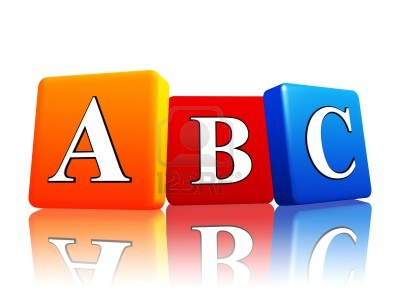### What is the Meaning of Dividend | Definition and What is DividendDividend, from the latin dividendus, is the quantity or number to divide by one or the other. In the arithmetic operation of division, he is trying to figure out how many times the divisor is contained in the dividend. The integer result of the division is called the quotient.
For example, if you divide 16 by 2 (16/2), we have the 2 as a divider and 16 as dividend. 8 is the result of the operation, i.e. the quotient.
It can therefore say that the dividend is equal to the quotient by the divider over the rest. In our previous example: 16 = 8 × 2 + 0.
In the account 45/5 = 9, 45 is the dividend, 5 is the divisor and 9 is the quotient (45 = 9 × 5 + 0).
In the field of economy and finance, the dividend is a return on investment that a company offers to its shareholders based on the number of shares of each. The dividend is paid with funds that come from the profits of the company for a certain period may be paid either in cash, or in more shares.
It belongs to the General Assembly of the shareholders of a company to decide when and how dividends are paid. If it is decided to pay 0.25 euros per share, any person who owns 5000 shares will receive 1250 euros. However, if the decision is to distribute shares to shareholders (dividends) and reward action on each 80 shares held, the person in the previous example will receive 62 shares (shares).